# Model parallelism with TensorFlow: sharding and pipelining¶

## Model parallelism¶

This technical note describes how to parallelise TensorFlow models on IPU hardware.

If a deep learning network has too many layers and parameters to fit on one IPU, we need to divide it into pieces and distribute those pieces across multiple IPUs. This is called the model parallelism approach, and it enables us to train large models that exceed the memory capacity on a single IPU accelerator. Currently, we support two types of model parallelism, Sharding and Pipelining.

## Sharding¶

Sharding means partitioning a neural network, represented as a computational graph, across multiple IPUs, each of which computes a certain part of this graph.

For example, we plan to train a model on a single Dell DSS8440 server (see DSS8440 Server white paper) that has a total of eight C2 cards (Graphcore C2 card) and 16 IPUs. As shown in the figure below, the model we are training has 32 layers that cannot fit in one IPU. Logically, we shard the model into 16 subgraphs across these eight C2 cards, where each IPU computes two subgraphs. The direction of the arrows indicates the computation process of the entire model. IPUs communicate with each other through IPU-Links, with bidirectional bandwidth of 64 GB/s.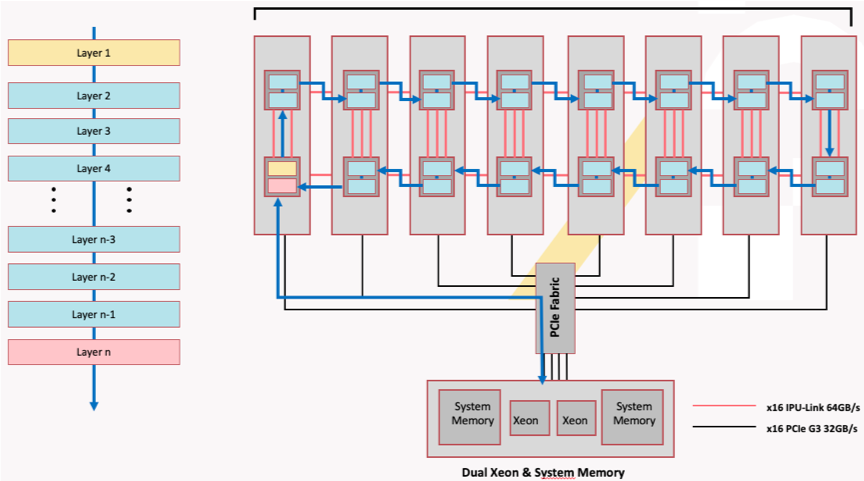Model sharding on Dell DSS8440 IPU server

The figure below shows how we shard a neural network that is implemented in TensorFlow. Even though this model is evenly partitioned across two IPUs and each subgraph can only be visible to its assigned IPU, these two subgraphs are encapsulated within the same session instance and therefore can be trained in a distributed manner.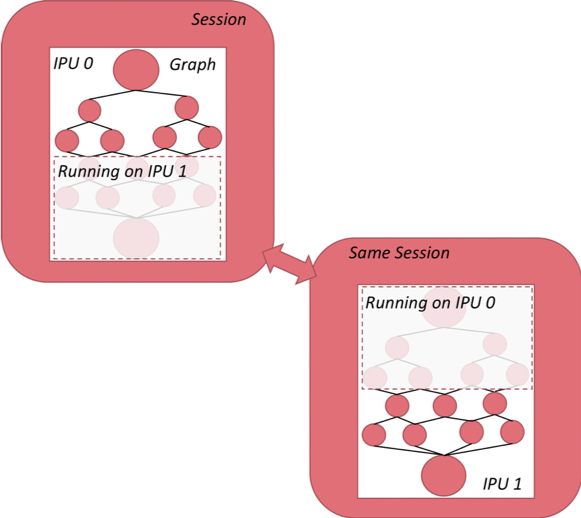Graph sharding with TensorFlow

In the figure below, on the left is the computational graph we would like to execute. Let’s say we assign a part of the graph (P0, P1, P2, P3) to the CPU, and partition the rest into two shards across two IPUs. The original computational graph (shown on the left) is transformed into the graph on the right. When the variables required for computation in TensorFlow are distributed on different types of TensorFlow devices (such as CPU and IPU), TensorFlow will add Send and Recv nodes to the graph. If we use sharding, copy nodes will be added between pairs of IPU shards to exchange variables. Copy nodes are implemented with IPU-Link technology.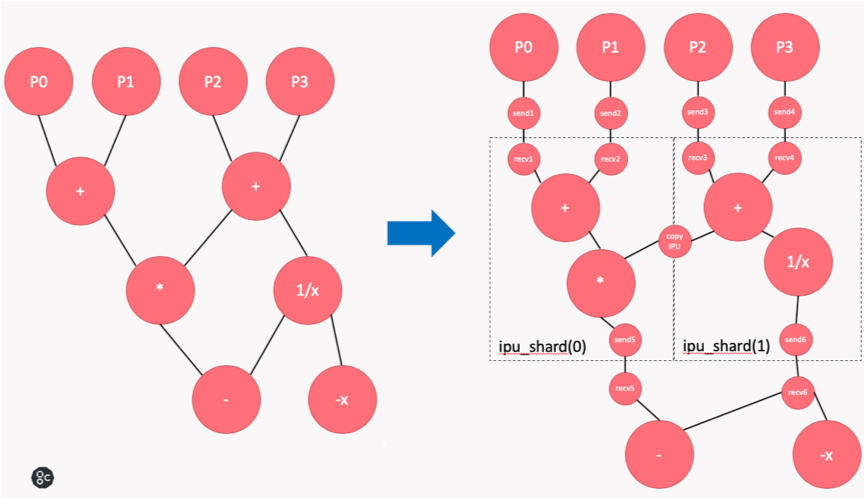Graph transformation with sharding

### Manual graph sharding¶

We provide an API for manual graph sharding, allowing you to arbitrarily designate sharding points of the graph to achieve maximum flexibility.

#### API¶

The manual model sharding API:

``` tensorflow.python.ipu.scopes.ipu_shard(index)
```

Model sharding of a group of operations.

Parameters:

• `index`: IPU index indicates which IPU the operation group is partitioned onto.

#### Code example¶

A code example is shown below:

 ``` 1 2 3 4 5 6 7 8 9 10 11 12 13 14 15 16 17 18 19 20 21 22 23 24 25 26 27 28 29 30 31 32 33 34 35 36 37 38 39 40 41 42 43 44 45 46 47 48 49 50 51 52``` ``` import numpy as np from tensorflow.python import ipu from tensorflow.python.ipu.scopes import ipu_scope from tensorflow.compiler.plugin.poplar.ops import gen_ipu_ops import tensorflow.compat.v1 as tf tf.disable_v2_behavior() NUM_IPUS = 4 # Configure the IPU system cfg = ipu.utils.create_ipu_config(profiling=True, use_poplar_text_report=True) cfg = ipu.utils.set_ipu_model_options(cfg, compile_ipu_code=False) cfg = ipu.utils.auto_select_ipus(cfg, NUM_IPUS) ipu.utils.configure_ipu_system(cfg) # Create the CPU section of the graph with tf.device("cpu"): pa = tf.placeholder(np.float32, , name="a") pb = tf.placeholder(np.float32, , name="b") pc = tf.placeholder(np.float32, , name="c") # Define a trace event with tf.device('cpu'): report = gen_ipu_ops.ipu_event_trace() # Distribute the computation across four shards def sharded_graph(pa, pb, pc): with ipu.scopes.ipu_shard(0): o1 = pa + pb with ipu.scopes.ipu_shard(1): o2 = pa + pc with ipu.scopes.ipu_shard(2): o3 = pb + pc with ipu.scopes.ipu_shard(3): out = o1 + o2 + o3 return out # Create the IPU section of the graph with ipu_scope("/device:IPU:0"): result = ipu.ipu_compiler.compile(sharded_graph, [pa, pb, pc]) with tf.Session() as sess: # sharded run result = sess.run(result, feed_dict={ pa: [1., 1.], pb: [0., 1.], pc: [1., 5.] }) print(result) ```

`auto_select_ipus` on Line 15 selects four independent IPUs for this task. This function browses the current IPUs in the system in order to determine which IPUs are idle and subscribes to four available IPUs. `sharded_graph()` on lines 29-38 defines a simple graph that consists of some simple additions. Most importantly, the entire graph is partitioned into four shards by calling the `ipu.scopes.ipu_shard()` API four times.

### Automatic graph sharding¶

In addition to manual graph sharding, we also provide an API for automatic graph sharding.

#### API¶

The API is:

``` tensorflow.python.ipu.autoshard.automatic_sharding(num_shards,
input_ts,
loss_ts,
edge_filter=None
frozen_inference=False)
```

Function to automatically partition the graph into N shards where N is specified by num_shards.

Parameters:

• `num_shards`: number of shards to split graph over.

• `input_ts`: tensor closest to the datafeed in the graph.

• `loss_ts`: tensor closest to the loss in the graph.

• `edge_filter`: a callable predicate, with the signature `fn(edge)`, where `edge` is a tuple with the name of the source operation, and the name of the destination operation.

• `frozen_inference`: flag set to `True` if running inference on a frozen graph. (Inference and training adopt different strategies when choosing the sharding point.)

Automatic-sharding algorithms are realised as follows:

• Analyse the forward graph to find all weakly connected edges. An edge can be determined as weakly connected when deletion of the edge leads to the entire digraph being shared into two independent weakly connected components (graph theory).

• Shard graph into N subgraphs through weakly connected edges.

• Calculate the estimated memory usage of each subgraph (estimation based on weight and activation).

• Combine some adjacent subgraphs to get `num_shards` subgraphs. The principle of this combining is to ensure that the memory of N subgraphs should be distributed in a uniform manner (the variance is the smallest).

Note

The automatic sharding algorithm has some limitations. The API is not applicable to graphs (such as BERT) that always have multilateral edges dependencies.

A code example is below:

 ``` 1 2 3 4 5 6 7 8 9 10 11 12 13 14 15 16 17 18 19 20 21 22 23 24 25 26 27 28 29 30 31 32 33 34 35``` ``` import numpy as np import tensorflow as tf from tensorflow.python.ipu import autoshard, ipu_compiler, scopes, utils NUM_SHARDS = 2 # With sharding all placeholders MUST be explicitly placed on # the CPU device: with tf.device("cpu"): pa = tf.placeholder(np.float32, , name="a") pb = tf.placeholder(np.float32, , name="b") pc = tf.placeholder(np.float32, , name="c") def basic_graph(pa, pb, pc): o1 = pa + pb o2 = pa + pc out = o1 + o2 return out def my_graph(pa, pb, pc): with tf.device("/device:IPU:0"): result = basic_graph(pa, pb, pc) autoshard.automatic_sharding(NUM_SHARDS, pa, result) return result out = my_graph(pa, pb, pc) fd = {pa: [1., 1.], pb: [0., 1.], pc: [1., 5.]} # Configure an IPU device that has NUM_SHARDS devices that we will # shard across. cfg = utils.create_ipu_config(profiling=True) cfg = utils.auto_select_ipus(cfg, NUM_SHARDS) utils.configure_ipu_system(cfg) with tf.Session() as sess: result = [sess.run(out, fd)] print(result) ```

This uses `auto_select_ipus` to select two independent IPUs for this task. `basic_graph()` defines a simple graph that consists of some simple additions. `my_graph()` automatically partitions the entire graph into two shards by calling the automatic sharding API.

### Limitations of sharding¶

Sharding technology simply partitions the model and distributes it on multiple IPUs. Multiple shards execute in series and cannot fully utilise the computing resources of the IPUs. As shown in the figure below, the model is partitioned into four parts and executed on four IPUs in series. Only one IPU is working at any one time.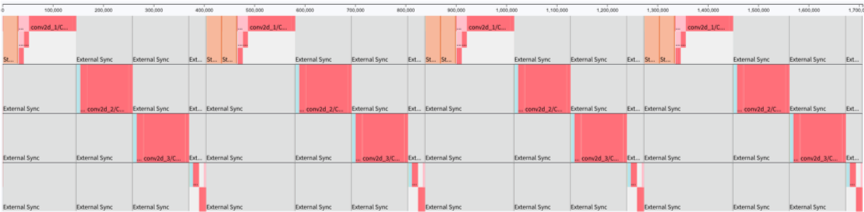Sharding profile

Sharding is a valuable method for use cases that need more control with replication, for example random forests, federated averaging and co-distillation. It can also be useful when developing/debugging a model, however for best performance pipelining should be used in most cases.

## Pipelining¶

### Overview¶

The pipeline approach is similar to sharding. The entire model is partitioned into multiple computing stages, and the output of a stage is the input of the next stage. These stages are executed in parallel on multiple IPUs. Compared to using sharding technology alone, the pipeline approach can maximise the use of all IPUs involved in parallel model processing, which improves processor efficiency as well as throughput and latency performance.

The figure below shows how to use pipelining for derivation in model parallelism (the dotted-line box indicates the point in the pipeline body where all IPUs are used to the maximum extent). The model consists of four layers and these are divided into four stages. Each stage is assigned to an IPU which computes a layer. When the first IPU receives a batch of data B1 and the first stage is executed, the second IPU starts to execute the second stage and, at the same time, the first IPU receives the next batch of data B2 and starts to execute the first stage, and so on. When the fourth batch of data B4 is read, the parallelism of the four IPUs reaches 100%.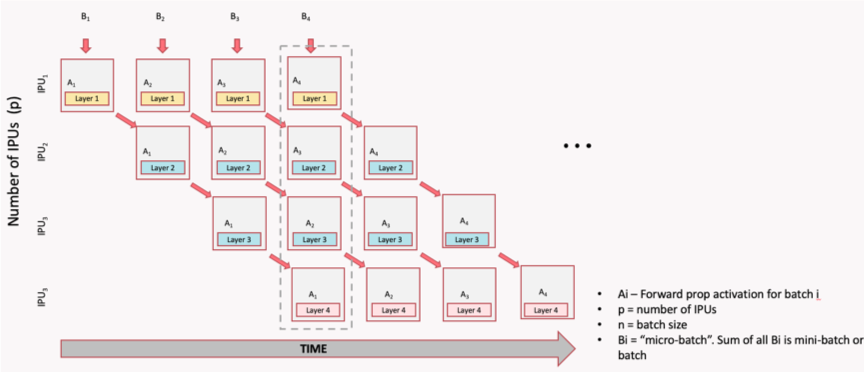Pipeline time sequence during model inference

The pipeline is relatively simple for inference, but more complicated for training based on back propagation. For training, pipelining needs to adapt to include forward pass, back propagation and weight update.

The figure below shows a single computational flow of forward pass and back propagation, and then shows a complete pipeline with parallel overlapping batches.

Each IPU performs not only the forward computation (Ai) of the corresponding layer, but also the gradient computation (AiGi). The dotted-line box shows the main body of the pipeline (it can be any depth, and larger depth can increase the size of the batch). Through the use of recomputation (see Optimising the pipeline), the relevant IPU is used to the maximum extent to process forward activations, the previous activations are recomputed from the stored activation inputs, and the gradient updates are computed to save valuable on-chip memory.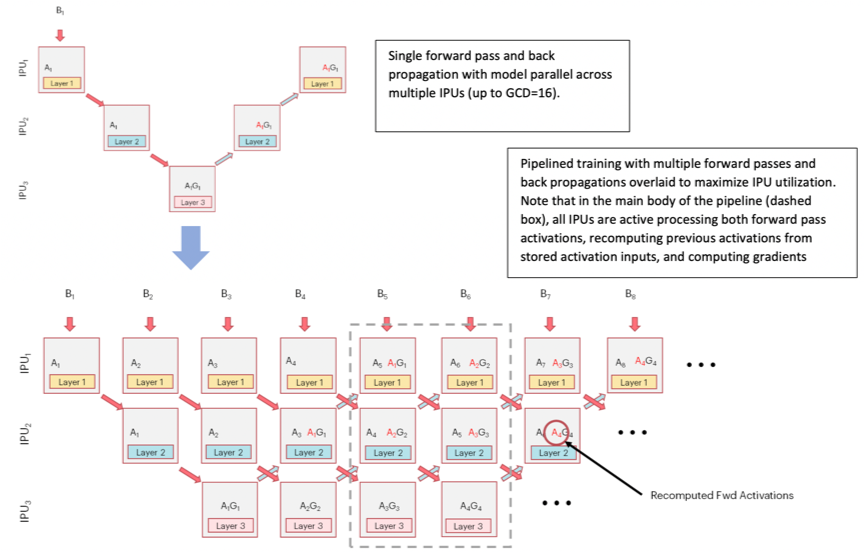Pipeline time sequence during model training

### Pipeline operation¶

There are three phases to the pipelined execution:

• Ramp up: this is the period in which the pipeline is being filled until every pipeline stage (including forward and backward passes) is performing computation. The maximum utilisation is 50%.

• Main execution: the time when all the pipeline stages are performing computation. This is the period when maximum use is being made of all the IPUs.

• Ramp down: the time when the pipeline is being drained until each pipeline stage is no longer performing any computation. The maximum utilisation is again 50%.

After ramp down, the weight updates are performed.

Note

Pipelining must not be combined with sharding.

### Pipelining API¶

The pipelining API allows the you to describe what the forward, backward and weight update operations are. You define the forward stages. The backward stages and the weight updates are automatically generated.

The pipelining interface is shown below.

 ``` 1 2 3 4 5 6 7 8 9 10 11 12 13 14 15``` ```  def pipeline(computational_stages, pipeline_depth, repeat_count=1, inputs=None, infeed_queue=None, outfeed_queue=None, optimizer_function=None, device_mapping=None, pipeline_schedule=None, forward_propagation_stages_poplar_options=None, backward_propagation_stages_poplar_options=None, weight_update_poplar_options=None, continuous_weight_updates=False, outfeed_loss=False, name=None): ```

Parameters:

• `computational_stages`: a list of Python functions, where each function represents a computational pipeline stage. The function takes the outputs of the previous pipeline state as its inputs.

• `pipeline_depth`: the number of times each pipeline stage will be executed or, equivalently, the number of mini-batches which will be consumed during a single execution of a pipeline. If the model you are using is defined as using a batch size B and the pipeline depth is P, this results in an effective batch size of B x P. This is important for convergence and optimiser choice.

• `repeat_count`: the number of times the pipeline will be executed. This helps to amortise the cost of `session.run()`. It is equivalent to wrapping the pipeline operation in a repeat loop but more efficient because the pipelining function is able to do more optimisations.

• `inputs`: arguments passed to the first pipeline stage.

• `infeed_queue`: optional `IPUInfeedQueue`, if passed, it is dequeued and passed as an input in the first pipeline stage.

• `outfeed_queue`: `IPUOutfeedQueue`; required if the last computational stage has any outputs. The outputs of these are enqueued to this queue and they can be accessed on the host.

• `optimizer_function`: optional Python function which takes the output of the last computational stage as parameters and returns an instance of `pipelining_ops.OptimizerFunctionOutput` in order to generate the back-propagation and weight-update parts of the model suitable for training. This is a structure which contains a TensorFlow optimiser and the loss.

• `device_mapping`: If provided, a list of length equal to the number of computational stages. An element at index `i` in the list represents which IPU the computational stage `computational_stages[i]` should reside on. This can be used to make sure that computational stages which share TensorFlow variables (`tf.Variable`) are resident on the same IPU.

• Information on other parameters can be found in the TensorFlow API documentation.

#### Inputs and outputs¶

All tensors which are used in the pipeline that are not TensorFlow variables need to be explicitly passed as inputs to the pipeline. If the input passed in does not change value – for example, hyper-parameters – add them to the `inputs` argument.

If the input does change value with every execution of a pipeline stage – for example, batches of data – then create an `IPUInfeedQueue` and pass it to the `infeed_queue` argument. The `inputs` list and the `infeed_queue` are passed as inputs to the first pipeline stage.

After the initial pipeline stage, all the outputs of a pipeline stage N are passed as inputs to the pipeline stage N+1. If an output of a stage N is used by a stage N+M where M > 1, then that output will be passed through the stages in between.

If the last computational stage has any outputs – for example, loss or the prediction – then you will need to create an `IPUOutfeedQueue` and pass it to the `outfeed_queue` argument. All the outputs from the final computational stage are passed to the outfeed automatically.

#### Device mapping¶

By default, the pipeline stages will be assigned to IPU devices in an order which should maximise the utilisation of IPU-Links between consecutive pipeline stages.

If your model is not sequential you might want to change the assignment, depending on the communication pattern in your model.

Any TensorFlow variables can only be used by pipeline stages which are on the same IPU. You can use the device mapping API to assign pipeline stages which use the same variable to be on the same IPU.

#### Pipeline scheduling¶

You can choose the method used for scheduling the operations in the pipeline. The scheduling methods have different trade-offs in terms of memory use, balancing computation between pipeline stages (and therefore the IPUs), and optimisations that can be applied. They will also have different pipeline depths and therefore different ramp-up and ramp-down times. The differences are most significant when training and you may need to experiment to find which method works best for your model.

In the Grouped schedule the forward and backward stages are grouped together on each IPU. All IPUs alternate between executing a forward pass and then a backward pass.

In the Interleaved schedule each pipeline stage executes a combination of forward and backward passes.

Finally, there is a sequential schedule. This is the same as sharding a model: only one batch is ever “in-flight”. This may be useful when you cannot have a big batch size but want to make use of other pipeline features.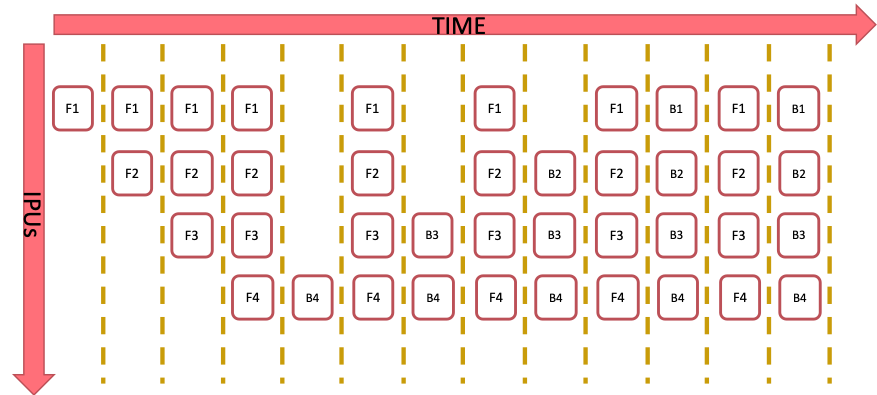Grouped schedule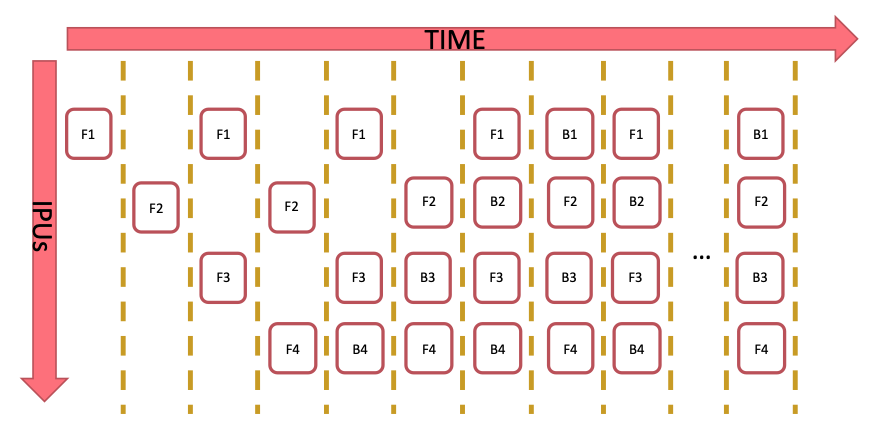Interleaved schedule

Memory use:

• The grouped schedule executes 2N batches at any given time.

• The interleaved schedule executes N batches.

• This means that the interleaved schedule requires less memory for the storing the data to be transferred between forward and backward passes.

Execution time:

• The grouped schedule executes all the forward stages together and all the backward stages together.

• The interleaved schedule executes the forward stages and backward stages interleaved.

• Due to the synchronisation required between stages, and the fact that the forward stages tend to use fewer cycles than the backward stages, the grouped schedule is likely to be faster.

Ramp-up and ramp-down time:

• The grouped schedule executes 2N batches in total to perform the ramp up and ramp down.

• The interleaved schedule executes N batches in total to perform the ramp up and ramp down.

Other:

• Some inter-IPU optimisations are not possible with the interleaved schedule. For example, an optimisation which converts variables which are passed through multiple pipeline stages into FIFOs.

### Code examples¶

#### Inference code examples¶

 ``` 1 2 3 4 5 6 7 8 9 10 11 12 13 14 15 16 17 18 19 20 21 22 23 24 25 26 27 28 29 30 31 32 33 34 35 36 37 38 39 40 41 42 43 44 45 46 47 48 49 50 51 52 53 54 55 56 57``` ``` from tensorflow.python import ipu from tensorflow.python.ipu import ipu_compiler from tensorflow.python.ipu import ipu_infeed_queue from tensorflow.python.ipu import ipu_outfeed_queue from tensorflow.python.ipu.ops import pipelining_ops from tensorflow.python.data.ops.dataset_ops import Dataset from tensorflow.python.ipu import scopes from tensorflow.python.ipu import utils from tensorflow.python.framework import ops from tensorflow.python.ops import variables from tensorflow.keras import layers import numpy as np import tensorflow.compat.v1 as tf tf.disable_v2_behavior() # default data_format is 'channels_last' dataset = Dataset.from_tensor_slices(np.random.uniform(size=(2, 128, 128, 3)).astype(np.float32)) dataset = dataset.batch(batch_size=2, drop_remainder=True) dataset = dataset.repeat() # Create the data queues from/to IPU. infeed_queue = ipu_infeed_queue.IPUInfeedQueue(dataset, "infeed") outfeed_queue = ipu_outfeed_queue.IPUOutfeedQueue("outfeed") # Create a pipelined model which is split accross two stages. def stage1(partial): partial = layers.Conv2D(128, 1)(partial) return partial def stage2(partial): partial = layers.Conv2D(128, 1)(partial) return partial def my_net(): pipeline_op = pipelining_ops.pipeline( computational_stages=[stage1, stage2], pipeline_depth=16, repeat_count=2, inputs=[], infeed_queue=infeed_queue, outfeed_queue=outfeed_queue, name="Pipeline") return pipeline_op with ops.device("/device:IPU:0"): r = ipu_compiler.compile(my_net, inputs=[]) cfg = utils.create_ipu_config() cfg = utils.auto_select_ipus(cfg, 2) utils.configure_ipu_system(cfg) utils.move_variable_initialization_to_cpu() with tf.Session() as sess: sess.run(variables.global_variables_initializer()) sess.run(infeed_queue.initializer) sess.run(r) ```

The code first creates a dataset with `infeed_queue` and `outfeed_queue` which are for data input and output. The functions `stage1()` and `stage2()` define two computation stages. The most important definitions are in `my_net()` which defines the entire behaviour of the pipeline. Among them, `computational_stages` indicates that the stage list contains `stage1` and `stage2`; `pipeline_depth=16` means that a pipeline is executed 16 times per stage, and `repeat_count=2` means that the pipeline is executed twice. The program selects two IPUs to perform this task using `auto_select_ipus()`, and each stage is automatically assigned to a single IPU.

### Training code examples¶

This example creates a pipeline of four stages with pipeline depth of 8 and a repeat count of 2. Four IPUs are selected for computation.

The selection order is ZIGZAG, and recomputation is enabled. The loss function is cross-entropy, and the optimiser is `tf.train.GradientDescentOptimizer()`.

The source code is shown below:

 ``` 1 2 3 4 5 6 7 8 9 10 11 12 13 14 15 16 17 18 19 20 21 22 23 24 25 26 27 28 29 30 31 32 33 34 35 36 37 38 39 40 41 42 43 44 45 46 47 48 49 50 51 52 53 54 55 56 57 58 59 60 61 62 63 64 65 66 67 68 69 70 71 72 73 74 75 76 77 78 79 80 81 82 83 84 85 86 87 88 89``` ``` from tensorflow.python import ipu from tensorflow.python.ipu import ipu_compiler from tensorflow.python.ipu import ipu_infeed_queue from tensorflow.python.ipu import ipu_outfeed_queue from tensorflow.python.ipu.ops import pipelining_ops from tensorflow.python.ops import variable_scope from tensorflow.python.data.ops.dataset_ops import Dataset from tensorflow.python.ipu import utils from tensorflow.python.framework import ops from tensorflow.python.ops import variables from tensorflow.keras import layers import numpy as np import tensorflow.compat.v1 as tf tf.disable_v2_behavior() # default data_format is 'channels_last' dataset = Dataset.from_tensor_slices( (tf.random.uniform([2, 128, 128, 3], dtype=tf.float32), tf.random.uniform(, maxval=10, dtype=tf.int32)) ) dataset = dataset.batch(batch_size=2, drop_remainder=True) dataset = dataset.repeat() # Create the data queues from/to IPU. infeed_queue = ipu_infeed_queue.IPUInfeedQueue(dataset, "infeed") outfeed_queue = ipu_outfeed_queue.IPUOutfeedQueue("outfeed") # Create a pipelined model which is split accross two stages. def stage1(partial, labels): with variable_scope.variable_scope("stage1", use_resource=True): with variable_scope.variable_scope("conv", use_resource=True): partial = layers.Conv2D(3, 1)(partial) return partial, labels def stage2(partial, labels): with variable_scope.variable_scope("stage2", use_resource=True): with variable_scope.variable_scope("conv", use_resource=True): partial = layers.Conv2D(3, 1)(partial) return partial, labels def stage3(partial, labels): with variable_scope.variable_scope("stage3", use_resource=True): with variable_scope.variable_scope("conv", use_resource=True): partial = layers.Conv2D(3, 1)(partial) return partial, labels def stage4(partial, labels): with variable_scope.variable_scope("stage3", use_resource=True): with variable_scope.variable_scope("flatten", use_resource=True): partial = layers.Flatten()(partial) with variable_scope.variable_scope("dense", use_resource=True): logits = layers.Dense(10)(partial) with variable_scope.variable_scope("entropy", use_resource=True): cross_entropy = tf.nn.sparse_softmax_cross_entropy_with_logits( labels=labels, logits=logits) with variable_scope.variable_scope("loss", use_resource=True): loss = tf.reduce_mean(cross_entropy) return loss def optimizer_function(loss): optimizer = tf.train.GradientDescentOptimizer(0.01) return pipelining_ops.OptimizerFunctionOutput(optimizer, loss) def my_net(): pipeline_op = pipelining_ops.pipeline( computational_stages=[stage1, stage2, stage3, stage4], pipeline_depth=8, repeat_count=2, inputs=[], infeed_queue=infeed_queue, outfeed_queue=outfeed_queue, optimizer_function=optimizer_function, name="Pipeline") return pipeline_op with ops.device("/device:IPU:0"): r = ipu_compiler.compile(my_net, inputs=[]) cfg = utils.create_ipu_config(selection_order=utils.SelectionOrder.ZIGZAG) cfg = utils.auto_select_ipus(cfg, 4) cfg = utils.set_recomputation_options(cfg) utils.configure_ipu_system(cfg) utils.move_variable_initialization_to_cpu() with tf.Session() as sess: sess.run(variables.global_variables_initializer()) sess.run(infeed_queue.initializer) sess.run(r) ```

Here, `tf.train.GradientDescentOptimizer()` automatically adds a stage to the pipeline for gradient computation, and a stage (`gradientDescent`) for weight update. Note that `pipeline_depth=8` means that `gradientDescent` is computed once every eight batches of data. And `repeat_count=2` means that the pipeline computes twice the `gradientDescent`; that is, the weight parameter is updated twice.

Execution can be traced using the profiler tool to get the execution information shown in the Training pipeline profile figure below.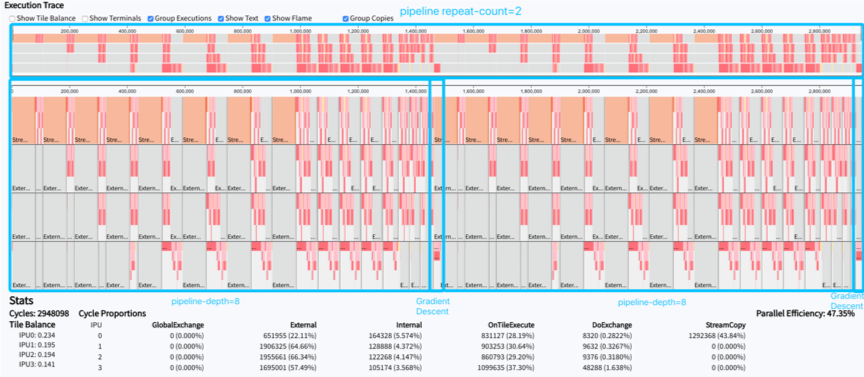Training pipeline profile

We can see from this figure that:

• The pipeline is repeated twice.

• A single pipeline repeat computes eight batches of data.

• Each batch of data goes through the phases of forward, gradient, and recomputation (optional).

• Four stages are executed in parallel on four IPUs.

• After eight gradient computations, a gradient descent will be executed, that is, the weight will be updated once.

### Optimising the pipeline¶

#### Recomputation¶

The Poplar SDK makes more efficient use of the valuable on-processor memory by saving selected activation inputs, optimising on memory savings vs TFLOP expenditure with recomputation. The two figures below demonstrate this, showing how the subset of activation inputs that are saved can be used to recompute all the necessary activation history for the backward pass calculation of the weight updates, thus saving on memory usage.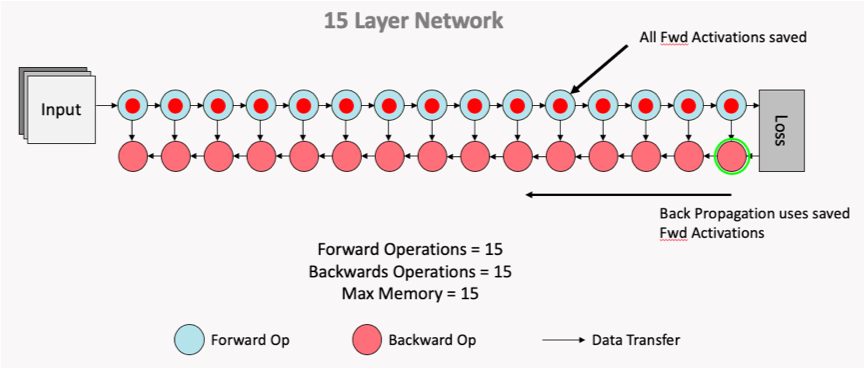Normal computation flow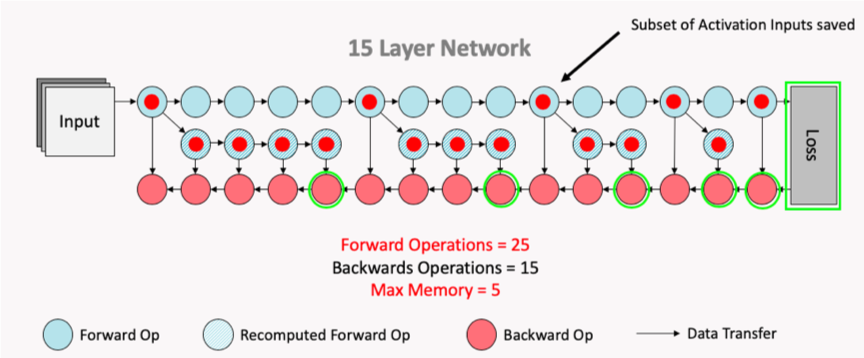Computation flow after recomputation enabled

#### Device selection order¶

Use the API to make sure the pipeline stage mapping to devices utilises the IPU-Links as much as possible.

#### Data parallelism¶

Pipelining supports replicated graphs. Use the standard `CrossReplicaOptimizer` in the optimiser function.

If the model you are working on is defined as using a batch size B and the pipeline depth is P and the replication factor is R, this results in an effective batch size of B x P x R.

Note that the all-reduce collectives for the gradients are only performed during the weight update.

#### Increase pipeline depth¶

The bigger the pipeline depth:

• The smaller proportion of time is spent during ramp up and ramp down.

• The smaller proportion of time is spent during a weight update.

#### Profiling¶

When your model is executing correctly, you can try moving layers around or changing the available memory proportion.

• Move layers towards the final computation stage to reduce the amount of recomputation

• Try to increase `availableMemoryProportion`. For example:

``` # Set "availableMemoryProportion" flag to "0.5"
opts = create_ipu_config()
opts = set_matmul_options(opts,
matmul_options={"availableMemoryProportion": "0.5"}) ipu.utils.configure_ipu_system(opts)
```
• More fine-grained control of the available memory proportion with the following options:

• `forward_propagation_stages_poplar_options`: If provided, a list of length equal to the number of computational stages. Each element is a `PipelineStageOptions` object which allows for fine grain control of the Poplar options for a given forward propagation computational stage

• `backward_propagation_stages_poplar_options`: If provided, a list of length equal to the number of computational stages. Each element is a `PipelineStageOptions` object which allows for fine grained control of the Poplar options for a given backward propagation computational stage

• `weight_update_poplar_options`: If provided, a `PipelineStageOptions` object which allows for fine grained control of the Poplar options for the weight update stage.

This can be useful in certain situations, for example if one stage is almost out of memory then the available memory proportion can be lowered there but not for the rest of the model.

• Make sure that the `tf.Dataset` passed to the pipeline is not the bottleneck. See the Dataset benchmarking section in Targeting the IPU from TensorFlow for more information.

• Experiment with Poplar engine options. For example:

``` POPLAR_ENGINE_OPTIONS='{"opt.enableSwSyncs": ”true"}'
```

## PopVision™ Graph Analyser tool¶

You can use the PopVision Graph Analyser tool to debug IPU programs and generate reports on compilation and execution of the program. This tool is included with Poplar SDK version 1.1 and can be downloaded from the Graphcore customer support portal: https://downloads.graphcore.ai/. There is a built-in help system within the tool for any questions you might have about producing and analysing reports.

PopVision Graph Analyser tool output below shows a PopVision Graph Analyser report generated by a pipeline example of forward inference computation.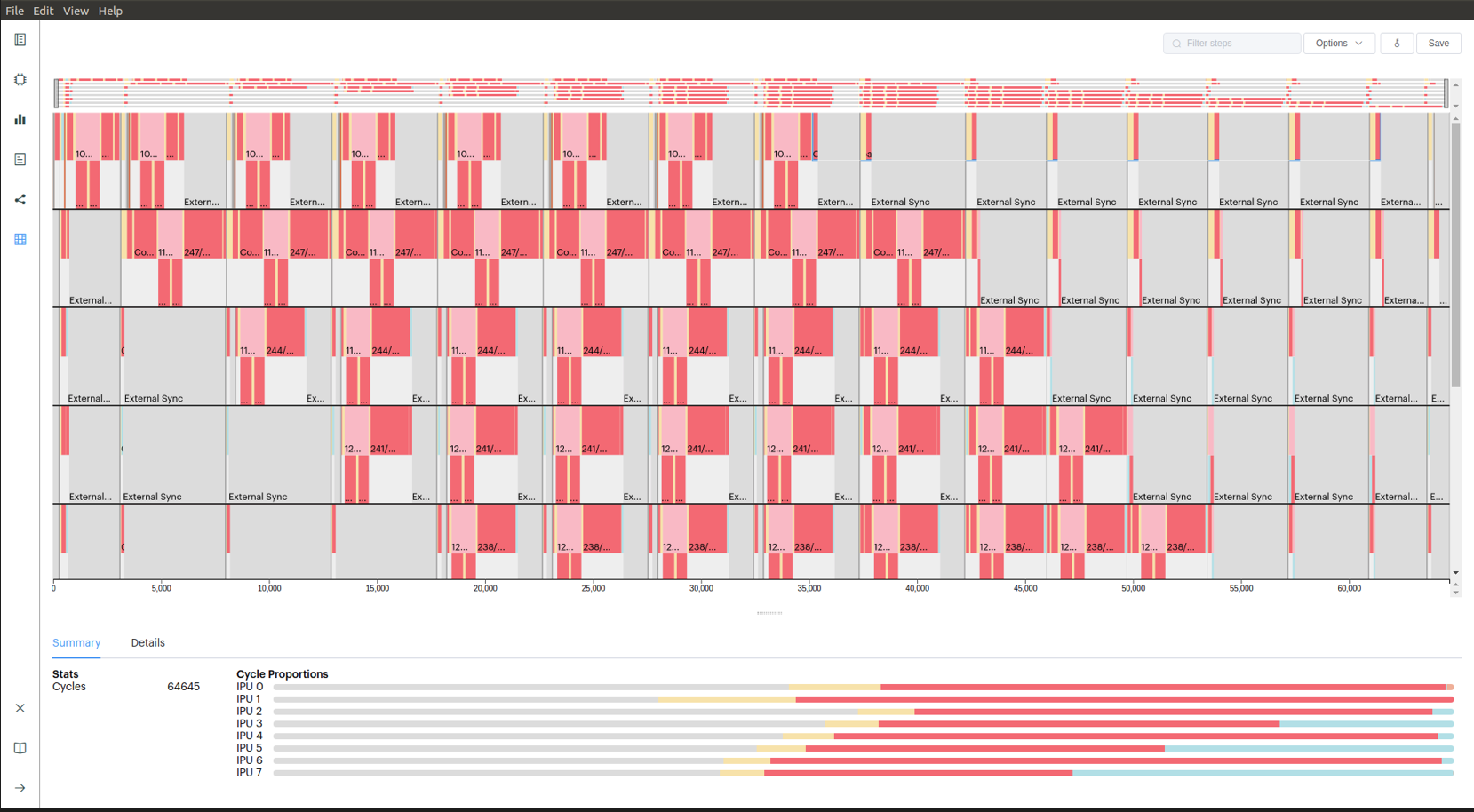PopVision Graph Analyser tool output